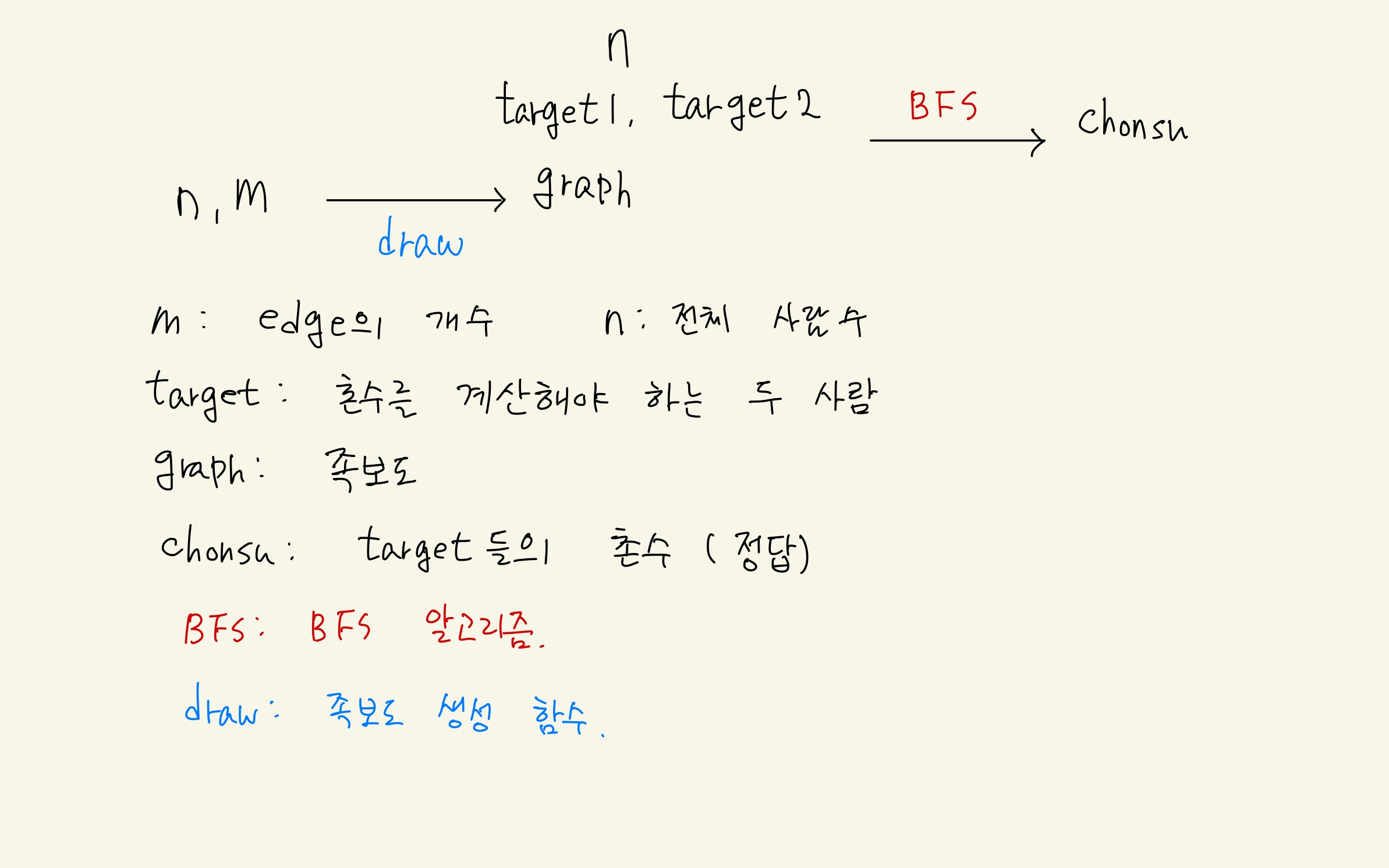# 촌수계산

happyyeon·2023년 1월 25일
0

## CodingTest

목록 보기
109/119촌수계산

# 설계도# 코드

from collections import deque
def draw(n,m):
graph = [[]*(n+1) for _ in range(n+1)]
for i in range(m):
a,b = map(int,input().split())
graph[a].append(b)
graph[b].append(a)
return graph
def bfs(n,target1,target2,graph):
q = deque([(target1,0)])
visited = [False]*(n+1)
visited[target1] = True

while q:
cur, chonsu = q.popleft()
if cur == target2:
return chonsu
for next in graph[cur]:
if not visited[next]:
q.append((next,chonsu+1))
visited[cur] = True
chonsu = -1
return chonsu

n = int(input())
target1,target2 = map(int,input().split())
m = int(input())
graph = draw(n,m)
chonsu = bfs(n,target1,target2,graph)
print(chonsu)